# How to extract number from string in Excel

The tutorial shows how to extract number from various text strings in Excel by using formulas and the Extract tool.

When it comes to extracting part of a text string of a given length, Excel provides three Substring functions (Left, Right and Mid) to quickly handle the task. When it comes to extracting a number from an alphanumeric string, Microsoft Excel provides… nothing.

To get a number from a string in Excel, it takes a little ingenuity, a bit of patience, and a bunch of different functions nested into each other. Or, you can run the Extract tool and have the job done with a mouse click. Below you will find full details on both methods.

## How to extract number from the end of text string

When you have a column of alphanumeric strings where number always comes after text, you can use one of the following formulas to get it.

Important note! In the below formulas, the extraction is performed with the RIGHT and LEFT functions, which belong to the category of Text functions. The output of these functions is always text. In our case, the result will be a numeric substring, which in terms of Excel is also text, not number. If you need the result to be a number (that you can use in further calculations), wrap a corresponding formula into the VALUE function as shown in the first example.

### Formula 1. Get number from the right of a string

To extract number from a 'text-number' string, the first thing you need to know is where to start the extraction. So, let's determine the position of the first digit with this generic formula:

MIN(SEARCH({0,1,2,3,4,5,6,7,8,9},cell&"0123456789"))

We will dwell on the formula's logic a bit later. For now, simply replace cell with a reference to the cell containing the original string (A2 in our case), and enter the formula in any empty cell in the same row, say in B2:

`=MIN(SEARCH({0,1,2,3,4,5,6,7,8,9},A2&"0123456789"))`

Although the formula contains an array constant, it's a regular formula completed in the usual way by pressing the Enter key.

Once the position of the first digit is determined, you use the RIGHT function to extract the number. To find out how many characters should be extracted, you subtract the position of the first digit from the total length of the string, and add one to the result because the first digit is also to be included:

`=RIGHT(B2, LEN(A2)-B2+1)`

Where A2 is the original string and B2 is the position of the first digit.

The following screenshot shows the results: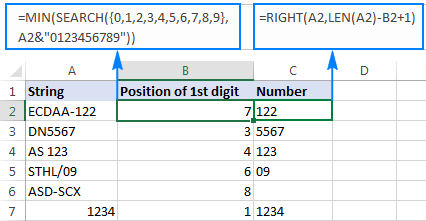To eliminate the helper column containing the position of the first digit, you can embed the MIN formula directly in the RIGHT function like this:

`=RIGHT(A2,LEN(A2) - MIN(SEARCH({0,1,2,3,4,5,6,7,8,9}, A2&"0123456789")) +1)`

To force the formula to return a number rather than a numeric string, nest it into the VALUE function:

`=VALUE(RIGHT(A2,LEN(A2) - MIN(SEARCH({0,1,2,3,4,5,6,7,8,9}, A2&"0123456789")) +1))`

#### How the position of the 1st digit is calculated

We supply the array constant {0,1,2,3,4,5,6,7,8,9} in the find_text argument of the SEARCH function, so that the formula searches for each element of the array within the original string and returns their positions. Because the array constant contains 10 digits, the resulting array also contains 10 elements.

The MIN function processes the resultant array and returns the smallest value, which corresponds to the position in of the first digit in the original string.

Additionally, we use a special construction (A2&"0123456789") to concatenate every possible number with the original string. In situations when a certain number in the array constant is not found within the source string, this construction acts like IFERROR forcing the formula to return a "fake" position equal to the string length +1 or more chars.  As the result, if the original string does not contain any number, like in row 7 in the screenshot above, the RIGHT formula returns an empty string.

To make things easier to understand, let's see how the formula works out for a specific cell, say A2. That cell contains the text string "ECDAA-05", for which the SEARCH function returns the following array {7,10,11,12,13,8,15,16,17,18}. Here's how:

• 0 is the 1st element of the array constant and the 7th character in the original string, therefore the first item of the resulting array is "7".
• 5 is the 6th element of the array constant and the 8th character in the original string, so the sixth item of the resulting array is "8".
•  No other item of the array constant is found in cell A2, and therefore the other 8 items of the resulting array represent the positions of corresponding digits in the concatenated string (ECDAA-050123456789).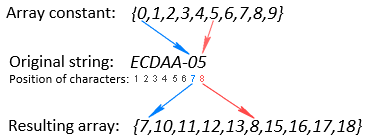Since 7 is the smallest value in the resulting array, the MIN function returns it, so we get the position of the first digit (0) in original string.

### Formula 2. Pull number from the right of a string

Another way to extract number from the end of a string is by using this generic formula:

RIGHT(cell,SUM(LEN(cell) - LEN(SUBSTITUTE(cell, {"0","1","2","3","4","5","6","7","8","9"},""))))

With the original text string in A2, you enter the below formula in B2 or any other empty cell in the same row, and then copy it down the column:

=RIGHT(A2,SUM(LEN(A2) - LEN(SUBSTITUTE(A2, {"0","1","2","3","4","5","6","7","8","9"},""))))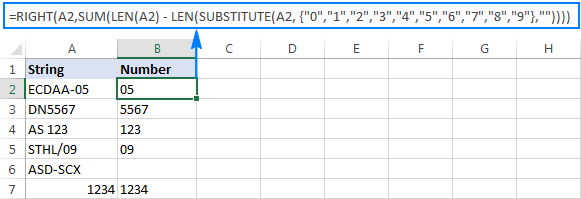#### How this formula works

In essence, the formula searches for all numbers from 0 to 9 within the source string, counts the found numbers, and returns that many characters from the end of the string.

And here is the detailed formula break down:

• First, you use the LEN and SUBSTITUTE functions to find out how many times a given number occurs in the string. For this, you replace the number with an empty string (""), and then subtract the length of the string without that number from the total length of the original string. This operation is performed on each number in the array constant.
• Next, the SUM function adds up all occurrences of all digits in the string.
• Finally, the digit count goes to the num_chars argument of the RIGHT function instructing it to return that many characters from the right side of the string.

## How to extract number from the beginning of text string

If you are working with strings where text appears after number, the formula for extracting number would be similar to the one discussed above, with the difference that you use the LEFT function to pull the characters from the left side of the string:

LEFT(cell,SUM(LEN(cell)-LEN(SUBSTITUTE(cell,{"0","1","2","3","4","5","6","7","8","9"},""))))

With the original string in A2, use the following formula to get number:

=LEFT(A2,SUM(LEN(A2)-LEN(SUBSTITUTE(A2,{"0","1","2","3","4","5","6","7","8","9"},""))))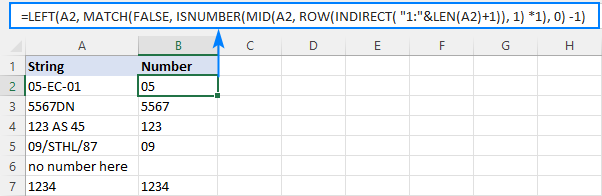Note. As is the case with the RIGHT function, the LEFT function returns a numeric substring, which is technically text, not number.

## How to get number from any position in a string

If your task implies extracting number from anywhere in a string, you can make use of the following mind-boggling formula published on MrExcel forum:

`=SUMPRODUCT(MID(0&A2, LARGE(INDEX(ISNUMBER(--MID(A2, ROW(INDIRECT("1:"&LEN(A2))), 1)) * ROW(INDIRECT("1:"&LEN(A2))), 0), ROW(INDIRECT("1:"&LEN(A2))))+1, 1) * 10^ROW(INDIRECT("1:"&LEN(A2)))/10)`

Where A2 is the original text string.

Breaking down this formula would require a separate article, so you can simply copy it to your worksheet to make sure it really works :)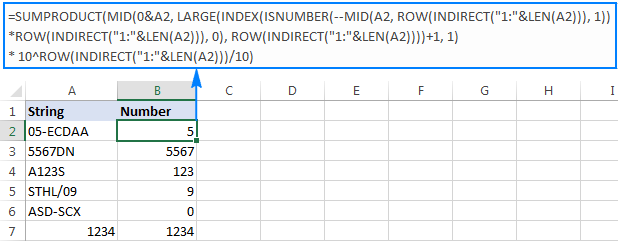Upon examining the results, however, you may notice one insignificant drawback - if the source string does not contain a number, the formula returns zero, as in row 6 in the screenshot above. To fix this, you can wrap the formula in the IF statement, the logical test of which checks if the source string contains any number. If it does, the formula extracts the number, otherwise returns an empty string:

`=IF(SUM(LEN(A2)-LEN(SUBSTITUTE(A2, {"0","1","2","3","4","5","6","7","8","9"}, "")))>0, SUMPRODUCT(MID(0&A2, LARGE(INDEX(ISNUMBER(--MID(A2,ROW(INDIRECT("\$1:\$"&LEN(A2))),1))* ROW(INDIRECT("\$1:\$"&LEN(A2))),0), ROW(INDIRECT("\$1:\$"&LEN(A2))))+1,1) * 10^ROW(INDIRECT("\$1:\$"&LEN(A2)))/10),"")`

As shown in the screenshot below, the improved formula works beautifully (kudos to Alex, our Excel guru, for this improvement):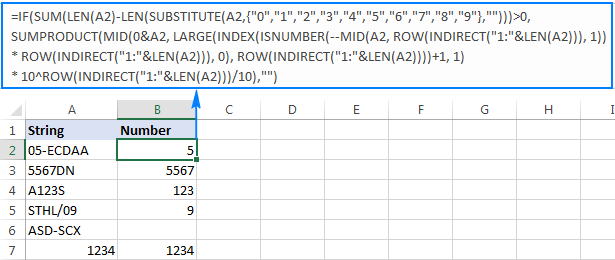Unlike in all previous examples, the result of this formula is number. To make sure of this, just notice the right-aligned values in column B and truncated leading zeros.

This is how you can extract number from string in Excel. To better understand and probably reverse-engineer the formulas, you are welcome to download our sample Excel Extract Number workbook.

## Extract number from text string with Ultimate Suite

As you have just seen, there is no trivial Excel formula to pull number from a text string. If you have difficulties with understanding the formulas or tweaking them for your data sets, you may like this simple way to get number from string in Excel.

With our Ultimate Suite added to your Excel ribbon, this is how you can quickly retrieve number from any alphanumeric string:

1. Go to the Ablebits Data tab > Text group, and click Extract: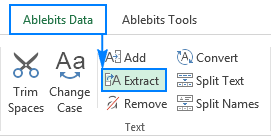2. Select all cells with the source strings.
3. On the Extract tool's pane, select the Extract numbers radio button.
4. Depending on whether you want the results to be formulas or values, select the Insert as formula box or leave it unselected (default).

My advice is to select this box if you want the extracted numbers to update automatically as soon as any changes are made to the source strings. If you want the results to be independent on the original strings (e.g. in case you plan to remove the source data at a later point), then do not select this box.

5. Click the Insert Results button. Done!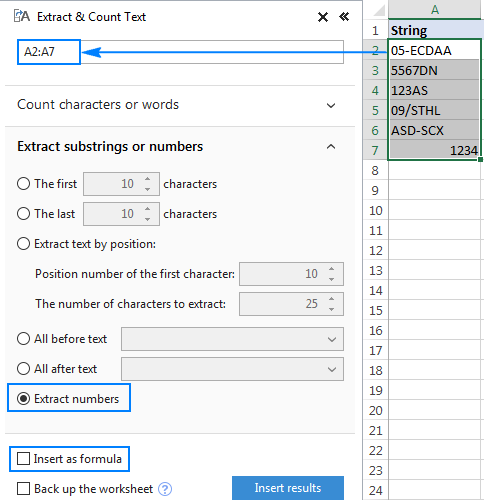Like in the previous example, the results of the extraction are numbers, meaning you are free to count, sum, average, or perform any other calculations with them.

In this example, we've chosen to insert the results as values, and the add-in did exactly what was asked for: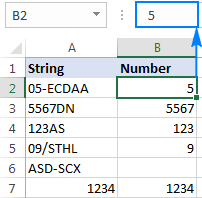If we selected the Insert as formula checkbox, you'd observe a formula in the formula bar. Curious to know which one? Just download Ultimate Suite's trial and see for yourself :)

If you'd like to have this as well as 60+ more useful tools in your Excel, be sure to grab the 15% off coupon code that we provide exclusively to our blog readers: AB14-BlogSpo.

I thank you for reading and hope to see you on our blog next week!

### 169 Responses to "How to extract number from string in Excel"

1.Sumit KK says:

Hey,
Svetlana Cheusheva
Please tell me how I can make a live input cell that is
"Underlying Index: NIFTY 11907.30 As on Jul 03, 2019 10:05:20 IST" in B1 CELL

to another cell say in C1 11907.30 and time in C2 10:50:20 to another cell.
or at least I can print C1 that would be also sufficient.

mainly viewed all the comment but not able to figure the amount

2.Brian B says:

This was immensely helpful, thanks. How do I get it to preserve any leading zeroes?

3.Brian B says:

I meant, how do I get the last method (extract from anywhere) to preserve leading 0's? Thanks.

4.Amit says:

Hi,
One thing, the SUBSTITUTE(A2,{"0","1","2","3","4","5","6","7","8","9"},"") doesn't seem to be working for me. It is not replacing the characters with empty string.
Data in cell A2: 25R
Expected: R

5.Cenzo Dontworryboutit says:

This is a great post.
thank you so much for your effort here. I wish my company wasn't so tight in the pockets so I could get all those add-ins

6.Dee says:

Ram mobile no-9925923457. Resides In Noida 119961
Can You suggest How To find Phn no. From Above Text

7.Deepak says:

Client Name
LALITA
GEETA DEVI NAYAK
MEHARUN NISHA
DIPA MANOJ
PREETI SINGHAL
meena devi swami
RAJIYA BEGAM
SHEHIDE
TARAWATI
BHATERI DEVI
sheela devi
JANKI DEVI
SUNITA
ALKA KANWAR
JAITUN
POOJA DEBI
CHHOTI DEVI
VIMLA DEVI
manju devi tak
MANJU
MUNNI DEVI
GEETA DEVI
TULSI DEVI
AILARAKHI
MUMTAJ BIBI
How to Find MID name if mid name more than 3 Character

8.sahara says:

The formula, of middle search doesnt fetch n give decimal place like 18.625

9.Fred Rogers says:

Not sure how to extract check no’s. From a text string having more that one set of numbers - see example below:

Brad James Company - Check - 23897 / invoice # 456755 issued Sept 1

Any suggestions are greatly appreciated - the line above is a sample of the excel items and after the check number shown there a number of invoice numbers in the text string.

Thanks , Fred

•Jayanth says:

If this is the data :
My Assumption is that all your data has "/" after the check number.
First :
Find the nth place of that "/" in that string using this formula :
=+FIND("/",D2)
Brad James Company - Check - 23897 / invoice # 456755 issued Sept 1
Output = 36
then,
use this formula =+MID(D2,C2-7,7)
here D2 is the input data which you have & C2 refers to the output of find formula i.e)36
then the output will be "23987".
Hope this helps! :)

10.Nilka F says:

This formula is exactly what I was looking for... and it works perfectly. Thank you so much! ;-)
=IF(SUM(LEN(A2)-LEN(SUBSTITUTE(A2, {"0","1","2","3","4","5","6","7","8","9"}, "")))>0, SUMPRODUCT(MID(0&A2, LARGE(INDEX(ISNUMBER(--MID(A2,ROW(INDIRECT("\$1:\$"&LEN(A2))),1))* ROW(INDIRECT("\$1:\$"&LEN(A2))),0), ROW(INDIRECT("\$1:\$"&LEN(A2))))+1,1) * 10^ROW(INDIRECT("\$1:\$"&LEN(A2)))/10),"")

11.Prashanth V says:

Hey,
Svetlana Cheusheva
Thank you so much for the tutorial. It is very nice of you. It was very helpful to me.

12.Kanchan says:

While this was very very helpful, I am facing a new kind of problem.
Job Id - #2416387528594195 is getting converted to '2416387528594190, excel is rounding off the last two digits and replacing with 0. Concat with an apostrophe also didn't help. I applied formula: =CONCAT("'",SUMPRODUCT(MID(0&B2,LARGE(INDEX(ISNUMBER(--MID(B2,ROW(INDIRECT("1:"&LEN(B2))),1))*ROW(INDIRECT("1:"&LEN(B2))),0),ROW(INDIRECT("1:"&LEN(B2))))+1,1)*10^ROW(INDIRECT("1:"&LEN(B2)))/10))

13.Sekar says:

This formula does not give the decimal values i.e 5.25, 7.3 and more. Kindly help me out on this.

Formula ,

IF(SUM(LEN(A2)-LEN(SUBSTITUTE(A2, {"0","1","2","3","4","5","6","7","8","9"}, "")))>0, SUMPRODUCT(MID(0&A2, LARGE(INDEX(ISNUMBER(--MID(A2,ROW(INDIRECT("\$1:\$"&LEN(A2))),1))* ROW(INDIRECT("\$1:\$"&LEN(A2))),0), ROW(INDIRECT("\$1:\$"&LEN(A2))))+1,1) * 10^ROW(INDIRECT("\$1:\$"&LEN(A2)))/10),"")

14.Kalyan Mondal says:

=SUM(MID(0&A3,LARGE(ISNUMBER(--MID(A3,ROW(INDIRECT("1:"&LEN(A3)))*ROW(INDIRECT("1:"&LEN(A3))),1)),ROW(INDIRECT("1:"&LEN(A3))))+1,1)*10^(ROW(INDIRECT("1:"&LEN(A3)))-1))

not getting any result if help if there is any error

15.Thomas says:

Thanks for the formulas (But I had trouble get them working. Here is why)
In some countries, Sweden among them, the "," character is a decimal delimiter. There for "SEARCH({0,1,2,3,4,5,6,7,8,9},A2)" results in an error. So for us we have to use another character in the syntax, ";". So here is what worked for me:
SEARCH({0;1;2;3;4;5;6;7;8;9},A2)

Hope above saves some time for others!

16.Excel is Powerfull T says:

The article was such Helpful that I got rid of a work that could have required me to dedicate lots of minutes. Thank you all.

17.Johannes says:

How can add the numbers of this following examples, without sorting it fisrt
CIVUS0.35B
CIVUS1W
IA-CIVUS0.13W
TAVUS0.35SB
AVSSF2W/B
AVS3W/B

18.Paul Flores says:

hi i have a cell from vendor quotes that i receive. this is how i name the vendor quote.
Lighting Expression 11-20-19 (\$132,833)
Vendor name - date provided - dollar amount
i want to extract only the dollar amount which can vary up to 2 million.

19.Saurabh says:

Below formula working fine but some number have percent sign between the text,i want extract number with percent sign which have % Sign as well.

=IF(SUM(LEN(A2)-LEN(SUBSTITUTE(A2, {"0","1","2","3","4","5","6","7","8","9"}, "")))>0, SUMPRODUCT(MID(0&A2, LARGE(INDEX(ISNUMBER(--MID(A2,ROW(INDIRECT("\$1:\$"&LEN(A2))),1))* ROW(INDIRECT("\$1:\$"&LEN(A2))),0), ROW(INDIRECT("\$1:\$"&LEN(A2))))+1,1) * 10^ROW(INDIRECT("\$1:\$"&LEN(A2)))/10),"")

60+ professional tools for Excel